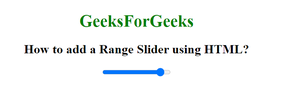# How to add a Range Slider using HTML ?

• Last Updated : 30 Sep, 2021

In this article, we will learn how to add a Range Slider in HTML. So Geeks as we know that a Range Slider is used to select a particular value from a range of numbers. We have default range values from 0 to 100.

Approach:

• First, we create an HTML document that contains a <form> element.
• Create an <input> element under the form element.
• Use the type attribute with the <input> element.
• Set the type attribute to value “range”.
• We can also use the min, max, step, and value attributes to set the restrictions on Range Slider.

Syntax:

`<input type="range">`

Example:

## HTML

 ```<``html``>`` ` `<``head``>``    ``<``title``>``        ``How to add a Range Slider using HTML?`` ` `    ```` ` `    ``<``style``>``        ``#Geek_p {``            ``font-size: 30px;``            ``color: green;``        ``}``    `````` ` `<``body` `style``=``"text-align:center;"``>`` ` `    ``<``h1` `style``=``"color:green;"``>``        ``GeeksForGeeks``    ```` ` `    ``<``h2``>``        ``How to add a Range Slider using HTML?``    ````    ``<``input` `type``=``"range"` `id``=``"Geek_Range"` `value``=``"90"``>```` ` ``

Output:My Personal Notes arrow_drop_up